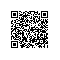# 神经网络初学者指南：基于Scikit-Learn的Python模块

## | 感知器| SciKit-Learn

## | 数据## | 数据预处理## | 训练模型

MLPClassifier(activation='relu', alpha=0.0001, batch_size='auto', beta_1=0.9,
beta_2=0.999, early_stopping=False, epsilon=1e-08,
hidden_layer_sizes=(30, 30, 30), learning_rate='constant',
learning_rate_init=0.001, max_iter=200, momentum=0.9,
nesterovs_momentum=True, power_t=0.5, random_state=None,
verbose=False, warm_start=False)

## | 预测与评估coefs_ 是权重矩阵的列表，其中索引i 处的权重矩阵表示层 i 和层 i + 1 之间的权重。

intercepts_ 是偏差向量的列表，其中索引i 处的向量表示添加到层 i + 1 的偏差值。

## | 结论

via python